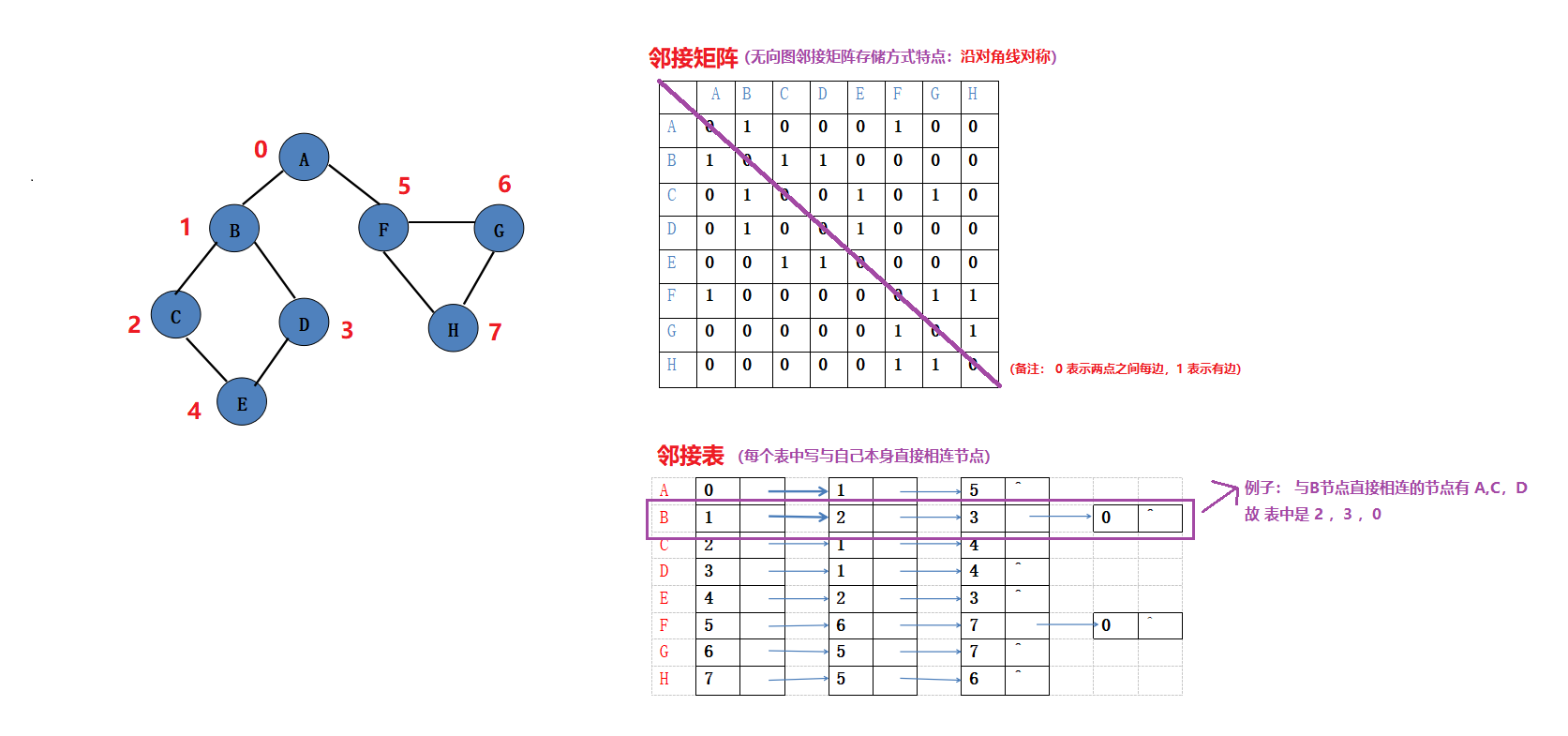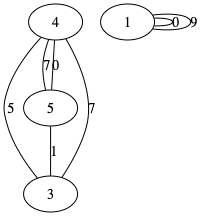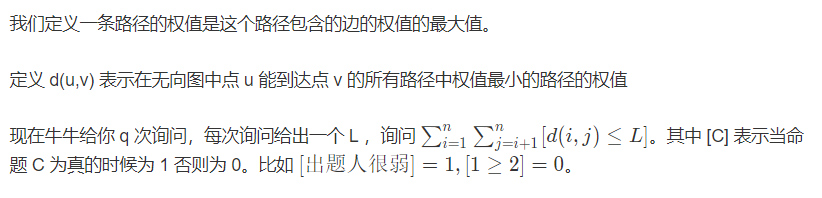• 求下图中无向图的邻接矩阵与邻接表储存方式及其DFS，BFS遍历 一、 无向图（无权值）的邻接矩阵存储方式及其DFS，BFS遍历 邻接矩阵的储存表示： 广度优先遍历需要用到队列操作，因此还需要定义一下队列的存储结构 ...
求下图中无向图的邻接矩阵与邻接表储存方式及其DFS，BFS遍历一、 无向图（无权值）的邻接矩阵存储方式及其DFS，BFS遍历
邻接矩阵的储存表示：广度优先遍历需要用到队列操作，因此还需要定义一下队列的存储结构具体代码：
#include <stdio.h>

#define MaxVex     100            //最大顶点数
#define TRUE       1
#define FALSE   0

typedef char    VertexType;    //顶点类型
typedef int     EdgeType;    //权值类型
typedef int     Bool;

Bool  visited[MaxVex];

typedef struct {
VertexType vexs[MaxVex];            //顶点数组
EdgeType   arc[MaxVex][MaxVex];    //邻接矩阵
int        numVertexes, numEdges;   //当前图中的结点数以及边数
}MGraph;

//广度优先遍历需要的循环队列
typedef struct {
int    data[MaxVex];
int    front, rear;
}Queue;

/****************************************/
//队列的相关操作

//初始化
void InitQueue(Queue *Q)
{
Q->front = Q->rear = 0;
}

//入队
void EnQueue(Queue *Q, int e)
{
if ((Q->rear+1)%MaxVex == Q->front)
return ;

Q->data[Q->rear] = e;
Q->rear = (Q->rear+1)%MaxVex;
}

//判空
Bool QueueEmpty(Queue *Q)
{
if (Q->front == Q->rear)
return TRUE;
else
return FALSE;
}

//出队
void DeQueue(Queue *Q, int *e)
{
if (Q->front == Q->rear)
return ;

*e = Q->data[Q->front];
Q->front = (Q->front+1)%MaxVex;
}
/****************************************/

//建立图的邻接矩阵
void CreateMGraph(MGraph *G){
int i, j, k;

printf("输入顶点数和边数: ");
scanf("%d%d", &G->numVertexes,&G->numEdges);

printf("==============================\n");
scanf("%c", &G->vexs[i]); /*关键，必须先要输入一次*/
printf("输入各个顶点:\n");
for (i=0; i< G->numVertexes; ++i){
scanf("%c", &G->vexs[i]);
}

for (i=0; i<G->numVertexes; ++i){
for (j=0; j<G->numVertexes; ++j)
G->arc[i][j] = 0;
fflush(stdin);
}

printf("==============================\n");
printf("输入边(vi, vj)中的下标i和j: \n");
for (k=0; k< G->numEdges; ++k){
scanf("%d%d", &i,&j);
G->arc[i][j] = 1;
G->arc[j][i] = G->arc[i][j];
}
}

/****************************************/
//图的深度优先遍历
void DFS(MGraph G, int i){
int j;
visited[i] = TRUE;
printf("%c ", G.vexs[i]);

for (j=0; j<G.numVertexes; ++j){
if (G.arc[i][j]!=0 && !visited[j])
DFS(G, j);
}
}

void DFSTraverse(MGraph G){
int i;
for (i=0; i<G.numVertexes; ++i)
visited[i] = FALSE;

for (i=0; i<G.numVertexes; ++i){
if (!visited[i])
DFS(G, i);
}

}

//图的广度优先遍历
void BFSTraverse(MGraph *G){
int i, j;
Queue Q;

for (i=0; i< G->numVertexes; ++i)
visited[i] = FALSE;
InitQueue(&Q);

for (i=0; i< G->numVertexes; ++i){
if (!visited[i]){
visited[i] = TRUE;
printf("%c ", G->vexs[i]);
EnQueue(&Q, i);

while (!QueueEmpty(&Q)){
DeQueue(&Q, &i);
for (j=0; j< G->numVertexes; ++j){
if (!visited[j] && G->arc[i][j]!=0){
visited[j] = TRUE;
printf("%c ", G->vexs[j]);
EnQueue(&Q, j);
}
}
}
}
}
}
/****************************************/

//程序入口
int main(){
MGraph G;

CreateMGraph(&G);

printf("\n图的深度优先遍历为: ");
DFSTraverse(G);

printf("\n图的广度优先遍历为: ");
BFSTraverse(&G);

printf("\n");

return 0;
}

结果展示：二、 无向图（无权值）的邻接表存储方式及其DFS，BFS遍历具体代码：
#include <stdio.h>
#include <stdlib.h>

#define MaxVertexNum 50 /*定义最大顶点数*/

typedef struct node {
/*边表节点*/
int adjVex;  /*邻接点域*/
struct node* next; /*链域*/
}EdgeNode;

typedef struct vNode {
char verTex; /*顶点域*/
EdgeNode *firstEdge; /*边表头指针*/
}verTexNode;

typedef verTexNode AdjList[MaxVertexNum]; /*AdjList是邻接表类型*/

typedef struct {
AdjList adjList; /*邻接表*/
int n,e; /*顶点数和边数*/
}ALGraph;

/*建立图的邻接表*/
void CreatALGraph(ALGraph *G) {
char a;
int i,j,k;
EdgeNode *s; /*定义边表节点*/
printf("Input VertexNum(n) and EdgesNum(e):");
scanf("%d,%d",&G->n,&G->e); /*输入顶点数和边数*/
scanf("%c",&a); /*避免 G->adjList.verTex = '\n' */
printf("Input Vertex string: ");
for (i = 0; i < G->n; ++i) {
scanf("%c",&a);
G->adjList[i].verTex = a; /*读入顶点信息*/
G->adjList[i].firstEdge = NULL; /*边表置空*/
}
printf("Input edges, Creat Adjacency List\n");
for ( k = 0; k < G->e; ++k) {
scanf("%d%d",&i,&j); /*读入边（Vi, Vj ） 的顶点对序号*/
s = (EdgeNode *) malloc(sizeof(EdgeNode)); /*生成边表节点*/
s->adjVex = j;
s->next = G->adjList[i].firstEdge;
G->adjList[i].firstEdge = s;
s = (EdgeNode *)malloc(sizeof(EdgeNode));
s->adjVex = i;
s->next = G->adjList[j].firstEdge;
G->adjList[j].firstEdge = s;
}
}

/*定义标志向量，为全局变量*/
typedef enum {FALSE, TRUE} Boolean;
Boolean visited[MaxVertexNum];

/*DFS遍历算法*/
void DFSM(ALGraph *G, int i){
EdgeNode *p;
printf("%c",G->adjList[i].verTex);
visited[i] = TRUE;
p = G->adjList[i].firstEdge;
while (p) {
if (!visited[p->adjVex])
DFSM(G, p->adjVex);
p = p->next;
}
}

void DFS(ALGraph *G){
int i;
for (i = 0; i < G->n; ++i) {
visited[i] = FALSE;
}
for ( i = 0; i < G->n; ++i) {
if (!visited[i]) {
DFSM(G,i);
}
}
}

/*BFS 遍历算法*/
void BFS(ALGraph *G, int k){
int i, f=0, r= 0;
EdgeNode *p;
int cQueue[MaxVertexNum]; /*定义FIFO队列*/
for (i = 0; i < G->n; ++i) {
visited[i] = FALSE;
}

for ( i = 0; i < G->n; ++i) {
cQueue[i] = -1; /*初始化标志向量*/
}
printf("%c", G->adjList[k].verTex);
visited[k] = TRUE;
cQueue[r] = k;
while (cQueue[f] != -1){
i = cQueue[f];
f++;
p = G->adjList[i].firstEdge;
while (p) {
if (!visited[p->adjVex]){
printf("%c", G->adjList[p->adjVex].verTex);
visited[p->adjVex] = TRUE;
r++;
cQueue[r] = p->adjVex;
}
p = p->next;
}
}
}

void main() {
ALGraph *G = (ALGraph *)malloc(sizeof(ALGraph));
CreatALGraph(G);
printf("Print Graph DFS:");
DFS(G);
printf("\n");
printf("Print Graph BFS:");
BFS(G,0);
}



结果展示：总结：

邻接矩阵是实现各种算法最简单的一种方式，其简单性主要来自数组读取元素的简洁性。但是其空间效率不高，适用于稠密图的存储。
邻接链表表示法，提高了空间的利用率，读取以某一节点为起点的所有边是比较简单的。但是链表的先天劣势就是随机读取方面需要移动指针，这就造成了算法的执行速度会比使用数组要慢许多。当然我们可以通过封装方法以及重载运算符的方式实现数组的功能，但是这只是代码层面的模仿，效率上是不可弥补的。除此之外，当要获得以某一节点为终点的所有边时，使用邻接表就显得十分无力了，算法实现显得十分笨拙：通过遍历所有节点的边链表，以检查是否存在满足条件的边。这里也突出了邻接矩阵的优势：它的随机读取方面的优势。
DFS,BFS遍历代码并不难理解，断点调试，分析代码运行过程，很快就能够理解。推荐工具： JetBrains公司旗下的C语言开发工具，Clion展开全文• 我们把有向图缩点为有向缩点树，则某一强连通块的权值就是该连通块下 所有正点权值和   这样我们就可以得到一个有向环图，显然我们选择起点是入度为0 点，因为所有入度不为0点 都能从别点走
题意：
给定n个点 m条有向边的图
每个点的点权
问：
遍历一遍图能得到的最大点权（对于经过的点，可以选择是否获得该点点权，但每个点只能被获得一次）
起点可以任意。

思路：
我们把有向图缩点为有向的缩点树，则某一强连通块的权值就是该连通块下的 所有正点权值和

这样我们就可以得到一个有向无环图，显然我们选择的起点是入度为0 的点，因为所有入度不为0的点 都能从别的点走过来。
因此我们建立虚根连接所有入度为0的点，然后跑一遍最长路spfa。

#include<stdio.h>
#include<string.h>
#include<iostream>
#include<vector>
#include<queue>
using namespace std;
#define inf 1000000
#define N 30100
//N为点数
#define M 150100
//M为边数
int n, m, a[N], val[N];

struct Edge{
int from, to, nex;
bool sign;//是否为桥
}edge[M<<1];
int head[N], edgenum;
void add(int u, int v){
Edge E={u, v, head[u], false};
edge[edgenum] = E;
head[u] = edgenum++;
}

int DFN[N], Low[N], Stack[N], top, Time;
int taj;//连通分支标号，从1开始
int Belong[N];//Belong[i] 表示i点属于的连通分支
bool Instack[N];
vector<int> bcc[N]; //标号从1开始

void tarjan(int u ,int fa){
DFN[u] = Low[u] = ++ Time ;
Stack[top ++ ] = u ;
Instack[u] = 1 ;

for (int i = head[u] ; ~i ; i = edge[i].nex ){
int v = edge[i].to ;
if(DFN[v] == -1)
{
tarjan(v , u) ;
Low[u] = min(Low[u] ,Low[v]) ;
if(DFN[u] < Low[v])
{
edge[i].sign = 1;//为割桥
}
}
else if(Instack[v]) Low[u] = min(Low[u] ,DFN[v]) ;
}
if(Low[u] == DFN[u]){
int now;
taj ++ ; bcc[taj].clear();
do{
now = Stack[-- top] ;
Instack[now] = 0 ;
Belong [now] = taj ;
bcc[taj].push_back(now);
}while(now != u) ;
}
}

void tarjan_init(int all){
memset(DFN, -1, sizeof(DFN));
memset(Instack, 0, sizeof(Instack));
top = Time = taj = 0;
for(int i=0;i<all;i++)if(DFN[i]==-1 )tarjan(i, i); //注意开始点标！！！
}
vector<int>G[N];
int du[N];
void suodian(){
memset(val, 0, sizeof(val));
for(int i = 1; i <= taj; i++)for(int j = 0; j < bcc[i].size(); j++)if(a[bcc[i][j]]>0)val[i] += a[bcc[i][j]];
memset(du, 0, sizeof(du));

for(int i = 1; i <= taj; i++)G[i].clear();
for(int i = 0; i < edgenum; i++){
int u = Belong[edge[i].from], v = Belong[edge[i].to];
if(u!=v)G[u].push_back(v), du[v]++;
}

}
int D[N];
bool inq[N];
int spfa(){
memset(inq, 0, sizeof(inq));
queue<int>q;
G.clear();
q.push(0);
D = 0;	val = 0;
for(int i = 1; i <= taj; i++){if(du[i] == 0)G.push_back(i); D[i] = -inf;}
int ans = 0;
while(!q.empty()){
int u = q.front(); q.pop(); inq[u] = 0;
for(int i = 0; i < G[u].size(); i++){
int v = G[u][i];
if(D[v] < D[u] + val[v])
{
D[v] = D[u] + val[v];
ans = max(ans, D[v]);
if(inq[v] == 0)inq[v] = 1, q.push(v);
}
}
}
return ans;
}
int main(){
int u, v, i, j;
while(~scanf("%d %d",&n,&m)){
memset(head, -1, sizeof(head)); edgenum = 0;
for(i = 0; i < n; i++)scanf("%d",&a[i]);
while(m--)scanf("%d%d",&u,&v), add(u,v);
tarjan_init(n);
suodian();
printf("%d\n",spfa());
}
return 0;
}
/*
2 2
14
21
0 1
1 0

*/
展开全文• 第一行包含两个整数N和 M， 表示该无向图中点数目与边数目。 接下来M 行描述 M 条边，每行三个整数Si，Ti ，Di，表示 Si 与Ti之间存在 一条权值为 Di无向边。 图中可能有重边或自环。 输出：仅包含一个整数，...
这道题要求从1到n的最大xor和路径，存在重边，允许经过重复点、重复边。
第一行包含两个整数N和 M， 表示该无向图中点的数目与边的数目。 接下来M 行描述 M 条边，每行三个整数Si，Ti ，Di，表示 Si 与Ti之间存在 一条权值为 Di的无向边。 图中可能有重边或自环。

输出：仅包含一个整数，表示最大的XOR和（十进制结果）

输入

5 7
1 2 2
1 3 2
2 4 1
2 5 1
4 5 3
5 3 4
4 3 2

输出

6
题目要求很清楚，看了大佬的博客，
不过还是自己手写一下思路吧。
可以从1道n随意找一条路径然后求出他的初始的异或和,作为初始值。
然后找到所有的环。。题解题解

我们考虑如何得到答案，首先所有的环都是可以经过的。这是为什么呢？
一个边权为非负整数的无向连通图,节点编号为1到N,试求出一条从1号节点到N号节点的路径,使得路径上经过的边得权值的XOR和最大.
假设我们从1号点开始走，走到一个环的起点，然后我们经过这个环以后回到了环的起点，这时我们可以直接回到起点。这样，除了环上的路径，其他的路径都被抵消了。

那么我们就只选了了这个环，也就是说，任意一个环都是可以选的。
然后我们先把所有的环都选出来，选入线性基中，再选出任意一条从1到n的路径，作为初始ans。初始ans异或线性基的最大值就是我们求的答案。为什么任意选一条路径也是可行的呢？
我们选了一条路径以后，如果存在一条更优的路径，那么这两条路径肯定是构成一个环的，会被选入线性基中。那么我们再用初始的ans异或一下这个环，我们就会发现，初始的ans被抵消了，二更优的那条路径留了下来。所以，我们选一个任意的初始ans是可行的。
于是这道题的实现就很明显了。先找出所有环，构成线性基，然后找出初始ans。这两步显然是可以dfs一遍一起搞的。然后用ans去异或线性基。从高位开始往低位异或。如果当前ans异或这一位的数能使ans变大，那么就异或。最终得到的ans就是我们要求的答案。

所以根据这题，我们得到一个结论：任意一条1到n的路径的异或和，都可以由任意一条1到n的路径的异或和和一些环的异或和来组合得到。

#include<bits/stdc++.h>
#include <iostream>
#include <cmath>
#include <cstdio>
#include <stdlib.h>
#include <ctime>
using namespace std;
typedef long long ll;
typedef pair<int,int> PII;
const int inf = 0x3f3f3f3f;
const int mod = 1e9+7;
const int maxn = 1e6 + 5;
using namespace std;
int n,m;
struct Point{
ll next,to,val;
}edge[maxn*2];
ll head[maxn],cnt;
ll cnn;//成环的个数
ll a[maxn];//线性基
ll A[maxn];//成环的点
ll d[maxn];//环中到i点的异或和
bool vis[maxn];//访问标记
void init(){
memset(head,0,sizeof(head));
memset(vis,false,sizeof(0));
memset(A,0,sizeof(A));
cnt=0;
cnn=0;
}
void add(ll u,ll v,ll w)
{
cnt++;
edge[cnt].to=v;
edge[cnt].val=w;
edge[cnt].next=head[u];
head[u]=cnt;
}
void dfs(int u)
{
vis[u]=true;
for(int i=head[u];i;i=edge[i].next)
{
int v=edge[i].to;
if(!vis[v])
{
d[v]=d[u]^edge[i].val;
dfs(v);
}
else
A[cnn++]=d[u]^d[v]^edge[i].val;//环的权值
}
}
void build(ll p)
{
for(int i=62;i>=0;--i)
{
if(p>>i&1)//if(p&ll(1ll<i))
{
if(a[i]==0)
{
a[i]=p;
break;
}
p^=a[i];
}
}
}
ll query_max()
{
ll ans=d[n];
for(int i=62;i>=0;--i)
{
if((ans^a[i])>ans)
ans^=a[i];
}
return ans;
}
int main()
{
ll u,v,w;
while(scanf("%d%d",&n,&m)!=EOF)
{
init();
for(int i=0;i<m;++i)
{
scanf("%lld%lld%lld",&u,&v,&w);
add(u,v,w),add(v,u,w);
}
dfs(1);
for(int i=0;i<cnn;++i)
build(A[i]);
ll ans=query_max();
cout<<ans<<endl;
}
return 0;
}


展开全文• 时间限制：C/C++ 1秒，其他语言2秒 ...定义 d(u,v) 表示在无向图中点 u 能到达点 v 所有路径中权值最小路径的权值 现在牛牛给你 q 次询问，每次询问给出一个 L ，询问 ∑i=1n∑j=i+1n[d(i,j)≤L]\sum_{i...


时间限制：C/C++ 1秒，其他语言2秒
空间限制：C/C++ 262144K，其他语言524288K
64bit IO Format: %lld

题目描述

牛牛有一张 n 个点，m 条边的无向图，每条边有一个边权 wiw_iwi​
我们定义一条路径的权值是这个路径包含的边的权值的最大值。
定义 d(u,v) 表示在无向图中点 u 能到达点 v 的所有路径中权值最小的路径的权值

现在牛牛给你 q 次询问，每次询问给出一个 L ，询问 ∑i=1n∑j=i+1n[d(i,j)≤L]\sum_{i=1}^n \sum_{j=i+1}^n [d(i,j) \leq L]∑i=1n​∑j=i+1n​[d(i,j)≤L]。其中 [C] 表示当命题 C 为真的时候为 1 否则为 0。比如 [出题人很弱]=1,[1≥2]=0[\text{出题人很弱}]=1,[1 \geq 2] = 0[出题人很弱]=1,[1≥2]=0。

为了防止输入过大，选手需要在自己的程序内生成要输入的所有数据，可以参考如下代码：

unsigned int SA, SB, SC; int n, m, q, LIM;
unsigned int rng61(){
SA ^= SA << 16;
SA ^= SA >> 5;
SA ^= SA << 1;
unsigned int t = SA;
SA = SB;
SB = SC;
SC ^= t ^ SA;
return SC;
}

void gen(){
scanf("%d%d%d%u%u%u%d", &n, &m, &q, &SA, &SB, &SC, &LIM);
for(int i = 1; i <= m; i++){
u[i] = rng61() % n + 1;
v[i] = rng61() % n + 1;
w[i] = rng61() % LIM;
}
for(int i = 1; i <= q; i++){
L[i] = rng61() % LIM;
}
}

生成的 ui,vi,wiu_i,v_i,w_iui​,vi​,wi​ 表示第 条边的属性，LiL_iLi​ 表示第 次询问输入的数。

输入描述:

一行七个正整数


输出描述:

一行，表示所有答案的异或和。

示例1

输入

复制5 7 5 480944053 701657892 339027200 10

5 7 5 480944053 701657892 339027200 10

输出

复制1

1

说明五次询问分别是 7，4，0，4，9

答案分别是 3，3，1，3，3

异或后答案是 1。

备注:

 

1≤n≤105,1≤m,q≤5×105,1≤LIM≤1091 \leq n \leq 10^5,1 \leq m,q \leq 5 \times 10^5,1 \leq LIM \leq 10^91≤n≤105,1≤m,q≤5×105,1≤LIM≤109

题目大意：输出所有询问的异或和。

解法：

首先MST能够使路径包含的边的权值最大值最小，这是容易观察到的性质，那么这个无向图就简化成了若干棵树。如果把树边都删掉，按照边权从小到大加回去，每条边的贡献恰好是两个点集大小的乘积，同时也能够满足第二条性质，这样求MST的过程中就能够解决所有的问题，剩下询问的答案可以离线也预处理前缀和再二分。

Accepted code

#pragma GCC optimize(3)
#include<bits/stdc++.h>
#include<unordered_map>
using namespace std;

#define sc scanf
#define Min(x, y) x = min(x, y)
#define Max(x, y) x = max(x, y)
#define ALL(x) (x).begin(),(x).end()
#define SZ(x) ((int)(x).size())
#define pir pair <int, int>
#define MK(x, y) make_pair(x, y)
#define MEM(x, b) memset(x, b, sizeof(x))
#define MPY(x, b) memcpy(x, b, sizeof(x))
#define lowbit(x) ((x) & -(x))
#define P2(x) ((x) * (x))

typedef long long ll;
const int Mod = 1e9 + 7;
const int N = 1e5 + 100;
const int M = 5e5 + 100;
const int INF = 0x3f3f3f3f;
const ll LINF = 0x3f3f3f3f3f3f3f3f;
inline ll dpow(ll a, ll b){ ll r = 1, t = a; while (b){ if (b & 1)r = (r*t) % Mod; b >>= 1; t = (t*t) % Mod; }return r; }
inline ll fpow(ll a, ll b){ ll r = 1, t = a; while (b){ if (b & 1)r = (r*t); b >>= 1; t = (t*t); }return r; }

unsigned int SA, SB, SC; int n, m, q, LIM;
unsigned int rng61(){
SA ^= SA << 16;
SA ^= SA >> 5;
SA ^= SA << 1;
unsigned int t = SA;
SA = SB;
SB = SC;
SC ^= t ^ SA;
return SC;
}
struct node
{
ll w;
int u, v;
bool operator < (const node &oth) const {
return w < oth.w;
}
}a[M];
int f[N], sz[N];
ll val[M], pre[M];

void Init() {
for (int i = 1; i <= n; i++)
f[i] = i, sz[i] = 1;
}
int Find(int x) {
while (x != f[x])
x = f[x];
return x;
}
void Merge(int x, int y) {
x = Find(x);
y = Find(y);
if (x == y)
return;
if (sz[x] > sz[y])
swap(x, y);
f[x] = y, sz[y] += sz[x];
}

int main()
{
sc("%d%d%d%u%u%u%d", &n, &m, &q, &SA, &SB, &SC, &LIM);
Init();
for (int i = 1; i <= m; i++) {
int u = rng61() % n + 1;
int v = rng61() % n + 1;
ll w = rng61() % LIM;
a[i] = { w, u, v };
}
sort(a + 1, a + m + 1);

int k = 0, pos = 0;
for (int i = 1; i <= m; i++) {
if (k == n - 1)
break;
int u = a[i].u, v = a[i].v;
if (Find(u) == Find(v))
continue;
pos++;
pre[pos] = pre[pos - 1] + 1ll * sz[Find(u)] * sz[Find(v)];  // 点对数前缀和
val[pos] = a[i].w;
Merge(u, v);
}

ll ans = 0;
for (int i = 1; i <= q; i++) {
int qi = rng61() % LIM;
int it = upper_bound(val + 1, val + pos + 1, qi) - val; // 二分位置
ans ^= pre[it - 1];
}
printf("%lld\n", ans);
return 0;  // 改数组大小!!!用pair记得改宏定义!!!
}


展开全文• /*（1）输入一组顶点，建立无向图的邻接矩阵。 进行DFS（深度优先遍历）和BFS（广度优先遍历）。 写出深度优先遍历的递归和非递归算法。*/ #include #define max 40 //最大顶点个数 #define M 10000 //无穷 #...
• 网络流说起最小割，最为朴素的算法大约是从网络流入手，枚举汇点，比较所得到的最大流（最大流=最小割），其中最小的就是我们所求的答案。 但是显然这样算是非常非常慢的，进行了很多次重复而毫无意义的计算。即便...算法
• 题意：给出无向图的点，边，权值。求最小割。   思路：根据题目规模，最大流算法会超时。 网上参考的模板代码。   代码： /*最小割集◎Stoer-Wagner算法:一个无向连通网络，去掉一个边集可以使其变成两个...
•  固定起点1和终点n，从1到n，再从n回到1，去和回路上相同边只能用一次，求两次和最短，如果去时候不能去到终点或者回时候回不到起点那么就输出Back to jail，否则输出两次和最小值（此图是无向图，不会...
• 题意：n个点m条边的无向连通图，起点s，每个点有权值，求遍历无向图得到的最大权值和。但是不能走回头路，即如果从U走到V那么下一步不可以从V走到U 题解：先缩点再跑个树形dp就可以了，无向图缩点还是第一次写，栈...
• /*（1）输入一组顶点，建立无向图的邻接矩阵。 进行DFS（深度优先遍历）和BFS（广度优先遍历）。 写出深度优先遍历的递归和非递归算法。*/ #include #define max 40 //最大顶点个数 #define M 10000 //无穷 #...
• 1.完整代码 ...#define MVNum 100 //最大顶点数 #define Max 20 typedef char VerTexType; //顶点数据类型为字符型 typedef int ArcType; //权值类型为整形 typedef int Status; typedef str算法 数据结构 dfs
• #include #include #include using namespace std; typedef char vertexType; //顶点数据类型，这里姑且设为字符，A,B,C,D ... //边信息，姑且定位权值 #define MAXVEX 100 //最大顶点数 bool visited[MAX
• 给你一个n个点，m条边的无向图，每一个点有两个属性ai,bi&lt;=INT_MAX 有q个询问，每一个询问，一个边界v,和k个点c1..ck 对于每一次询问，进行操作(此操作只作用于本次询问) 先将ai&gt;v点全部删除，...
• #include<stdio.h> #include<stack> #include <iostream> #define MAXSIZE 100 #define MaxInt 32767 //表示最大值，即正无穷大 #define MVNum 100 //定义最大顶点数...//假设边的权值为整型 type.
• 一开始看到这道题，想到的是最短路，用dijkstra，但是n的范围太大了，直接超内存，看了题解之后发现是并查集的思路。...答案就是所有边的最大权值。 #include<bits/stdc++.h> using namespace std; #define.
• 无向图的点连通度。解决办法：拆点，转换为求边连通度。通常情况下怎么求边连通度？给每条边赋权值1，任意选择源点，枚举汇点，依次求最小割。那么求无向图的点连通度该怎么建图？拆点，对于原始无向图中的边(a,b)...network 网络 ini 扩展
• 因为d(i,j)d(i,j)d(i,j)是iii到jjj所有路径中最大权值的最小值 那么我们建立最小生成树就可以最小化这条最大边权,不过还是没办法求… 考虑动态维护生成树 因为加边时候是小到大加边 当我们有一个查询xxx时,我们...
• 由于有n个点n-1条边 所以这个无向图可转化为一棵树 在树上距离为2位置关系有两种 ①距离他们LCA距离均为1 ② 其中一个点是另一个点父节点父节点 深搜 统计以每个节点为根节点子树最浅（除根节点外）两...
• //图的数组表示法 #define INFINITY INT_MAX //权值最大值 #define MAX_VERTEX_NUM 20 /...//{有向图,有向网,无向图,无向网} typedef struct ArcCell{//弧结点结构 VRType adj; //权值,无权图用1/0表示是否相邻 Inf
• 1、两点之间的行进路线，最终权值为所经过的边的权值的最大值 2、两点之间走法不止一个，最终取最小值为最终走法 问： 两点之间的最终权值为多少 如上，我们可以将其写为列表形式，前两位是从小到大的的两个点，...
• 第一行包含两个整数N和 M， 表示该无向图中点数目与边数目。 接下来M 行描述 M 条边，每行三个整数Si，Ti ，Di，表示 Si 与Ti之间存在 一条权值为 Di无向边。 图中可能有重边或自环。 Output 仅...
• 无向图最小点割集，确定起点S，终点T。每个点都有自己权值vi，求最小点权和割点集，使得S无法到达T。 解法：将每个点拆分为两个点v和v'，之间的权值为vi，将原图中每条边赋权值为INF（无穷大），然后使用...算法
• POJ 2914题意：给定一个无向图 小于500节点，和边的权值，求最小代价将图拆为两个联通分量。 Stoer Wagner算法： （1）用类似prim算法方法求“最大生成树”，但是它比较的权值为w(A,x)A为所有在树上点，x不...
• = 0，那么就可以在(u，v)之间连一条边，求最后图的最小环(环由几个点构成) 题解：逻辑运算 & 是二进制下的运算，题目给的每个权值 a[i] 的范围最大是1018，即二进制下最多64位。如果64位中有某一位的1的出现...
• [题目分析]连通图的生成树包括图中的全部n个顶点和足以使图连通的n-1条边，最小生成树是边上权值之和最小的生成树。...voidSpnTree (AdjList g)//用“破圈法”求解带权连通无向图的一棵最小代价生成树。{typ...
• 无向图最小点割集，确定起点S，终点T。每个点都有自己权值vi，求最小点权和割点集，使得S无法到达T。  解法：将每个点拆分为两个点v和v'，之间的权值为vi（单向边），将原图中每条边赋权值为INF（无穷大）...
• 题意： 给你中每两个点最短路，问你可不可以增加一条边的权值，使最小值不受影响，让这个最大最大。 思路：一个已经给定了，怎样才能增加一个边的权值使他最小值不受影响呢，应该可以想到就是当一个点可以...
• 1,v 寻找v所在连通块内权值最大的点，并输出这个权值并且把改点权值变成0 2.x 删掉第i条边 思路： 查询上连通块内最大值和修改我们只学过在一棵树上利用dfs序，然后线段树维护。 那么考虑如何将此题转化成一棵树。...
• 很多问题都可以转化为DAG上最长（短）路路径，最多（少）路径数（路径的权值为1） 对于状态d[i]设置可以有两种： 1.d[i]表示从i出发最长路 一般这种时候会考虑打印路劲，在出发之后会同时用一个数组来......